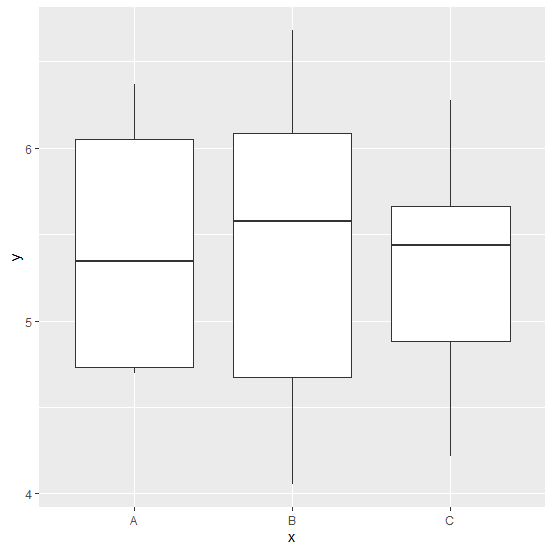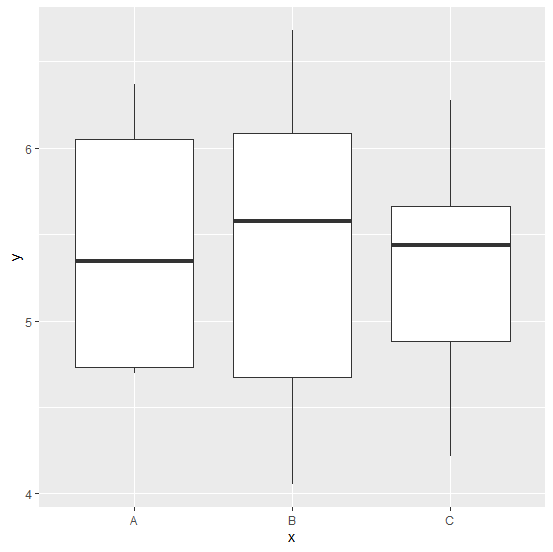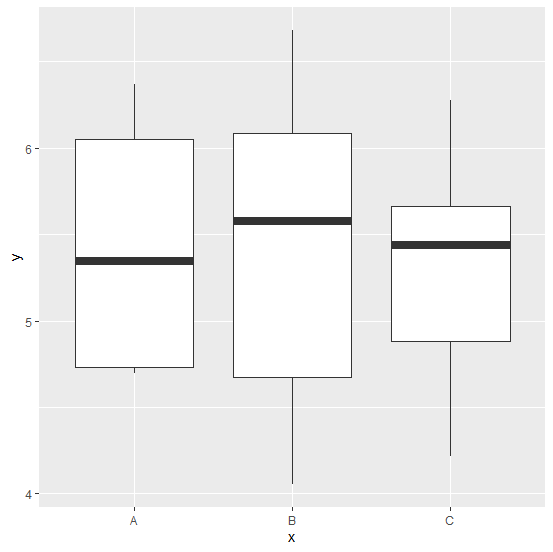# How to increase the width of the median line in boxplot using ggplot2 in R?

The default width of the median line is wider than the rest of the lines that represent minimum, first quartile, third quartile or maximum but we can make it a little wider to make it more appealing. This can be done with the help of fatten argument inside geom_boxplot function, the default value of fatten is 2.

## Example

Live Demo

Consider the below data frame −

x<−sample(c("A","B","C"),20,replace=TRUE)
y<−rnorm(20,5,1.24)
df<−data.frame(x,y)
df

## Output

   x    y
1 A 4.740834
2 C 5.365280
3 B 5.250932
4 B 5.764159
5 B 4.053119
6 B 4.241961
7 B 6.285087
8 C 5.513231
9 B 5.888086
10 B 4.479830
11 C 4.217874
12 A 6.366376
13 C 6.278470
14 C 4.721193
15 B 5.387542
16 B 6.683681
17 B 6.153023
18 A 4.695209
19 C 5.715360
20 A 5.948239

## Example

library(ggplot2)
ggplot(df,aes(x,y))+geom_boxplot()

## OutputCreating the boxplots with wider median lines −

## Example

ggplot(df,aes(x,y))+geom_boxplot(fatten=3)s

## Output## Example

ggplot(df,aes(x,y))+geom_boxplot(fatten=6)

## OutputUpdated on: 05-Feb-2021

547 Views